It is usually impractical to measure everything if you are trying to collect data. That is why you will use an appropriate sampling technique. But how big a sample should you get? Using a sample size calculator will make that task quick and easy. Let’s see how.

When you are required to collect data to answer a business question, it is usually not practical to collect the entire set of available data. As a result, you will want to utilize an appropriate sampling procedure such as random sampling, stratified random sampling, systematic, or periodic sampling.

There are standard formulas for calculating the size of the sample you would need to provide you with a certain level of accuracy and precision. The appropriate sample size calculations take into consideration the desired confidence level, precision, and are adjusted for the estimated variation of the population.

In some cases, the size of the population will also impact the sample size calculations. Whether you are collecting continuous or discrete data will also determine the relevant formula to use.

## Overview: What is a sample size calculator?

There is a plethora of sample size calculators on the Internet that you can just put in your desired values, and it will provide you the minimum required sample size. There are also several Excel based worksheets which you can use for calculating your required sample size.

Whether you are using an online sample size calculator, or an Excel based worksheet, you will need to provide the following information:

1. The estimated population size
2. Your desired confidence level (e.g. 90%, 95%, or 99%)
3. You desired precision or margin of error (e.g. 01, .05, .10)
4. The estimated standard deviation of the population if you are using continuous data

Below are two screen shots from an Excel based sample size calculator. The first is for continuous data and the second is for discrete data sample size calculations.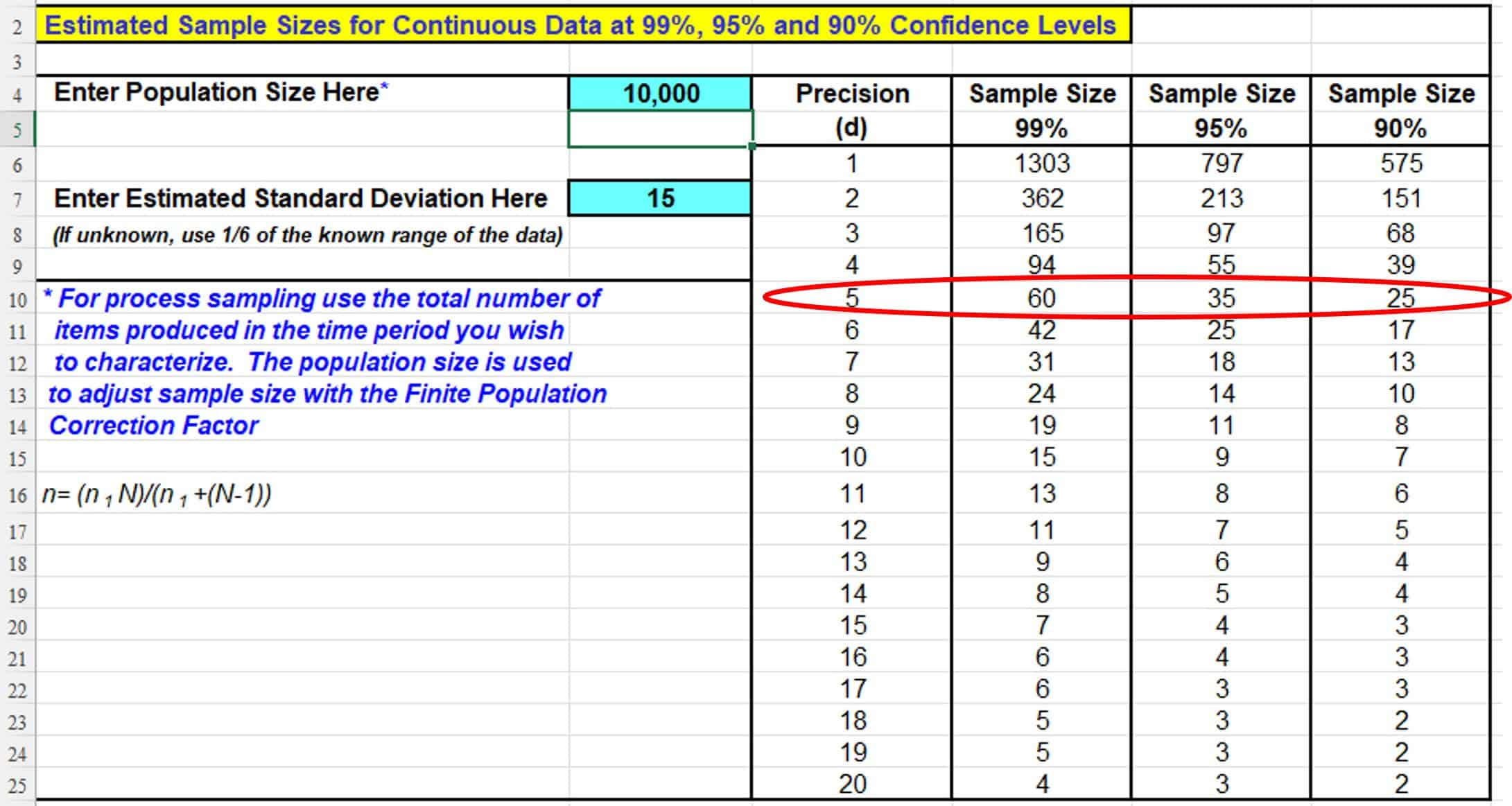Note, that for a population of 10,000, an estimated standard deviation of 15, and a desired precision of plus and minus 5, the minimum sample size is 60, 35, or 25 depending on your desired confidence level of 99%, 95%, or 90%.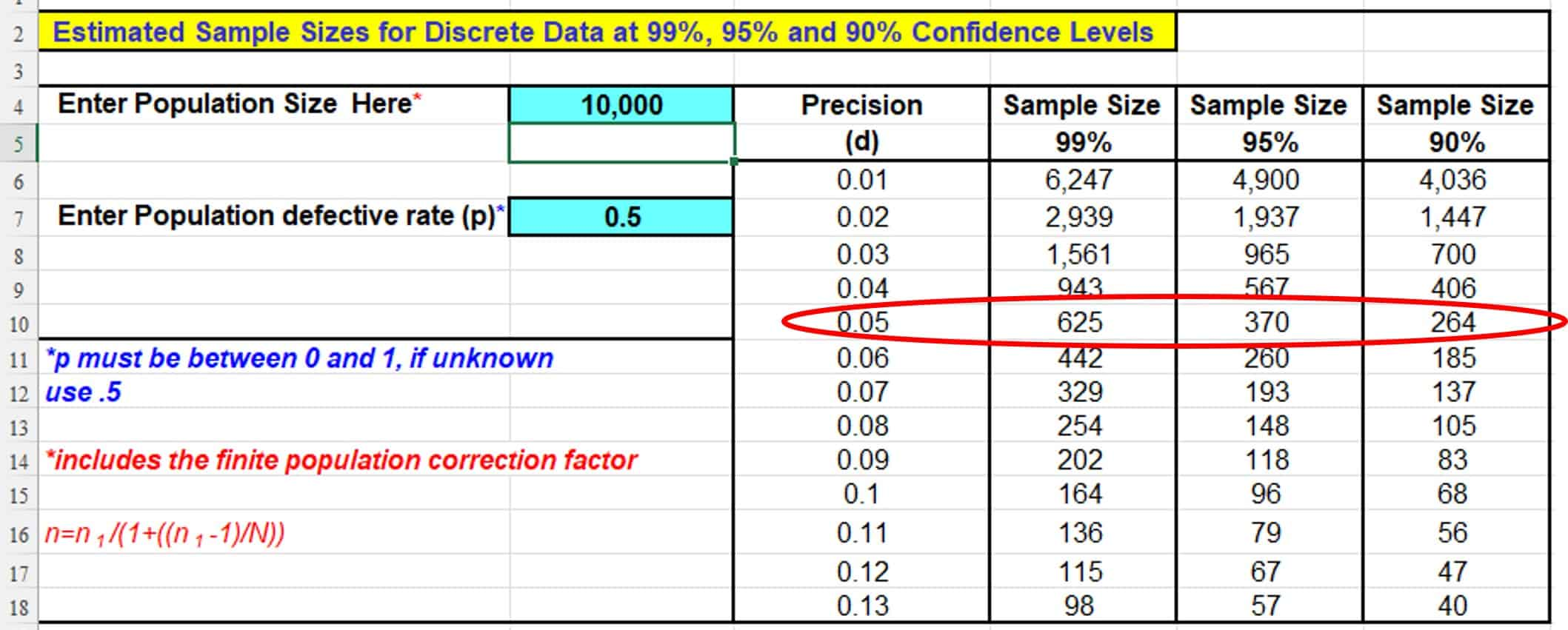Note that for a population of 10,000 and an estimated population defective rate of 50% (this is the default if this is unknown), and a desired precision of 5%, the minimum sample size is 625, 370, and 264 for confidence levels of 99%, 95%, and 90%.

Below is a screenshot of an online calculator for the same information as the discrete calculations above. Note the sample size calculation is the same.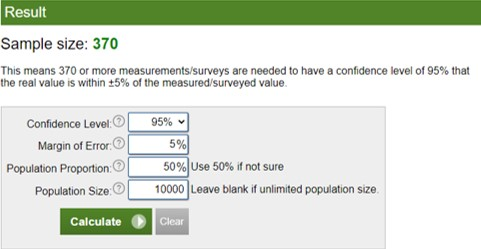Online sample size calculator

## An industry example of a sample size calculator

A small company wanted to survey a sample of their employees to understand how they felt about some newly implemented programs. The number of employees was 350 people. The company wanted a 95% confidence level and a 5% level of precision. Since they had no information on how the employees would respond, they used an estimate of 50% proportion.

Below is the standard sample size calculation. Notice that the recommended sample size of 385 exceeded the number of employees. As a result, they recalculated using the Finite Population Correction Factor to determine a more reasonable sample size of 184.

##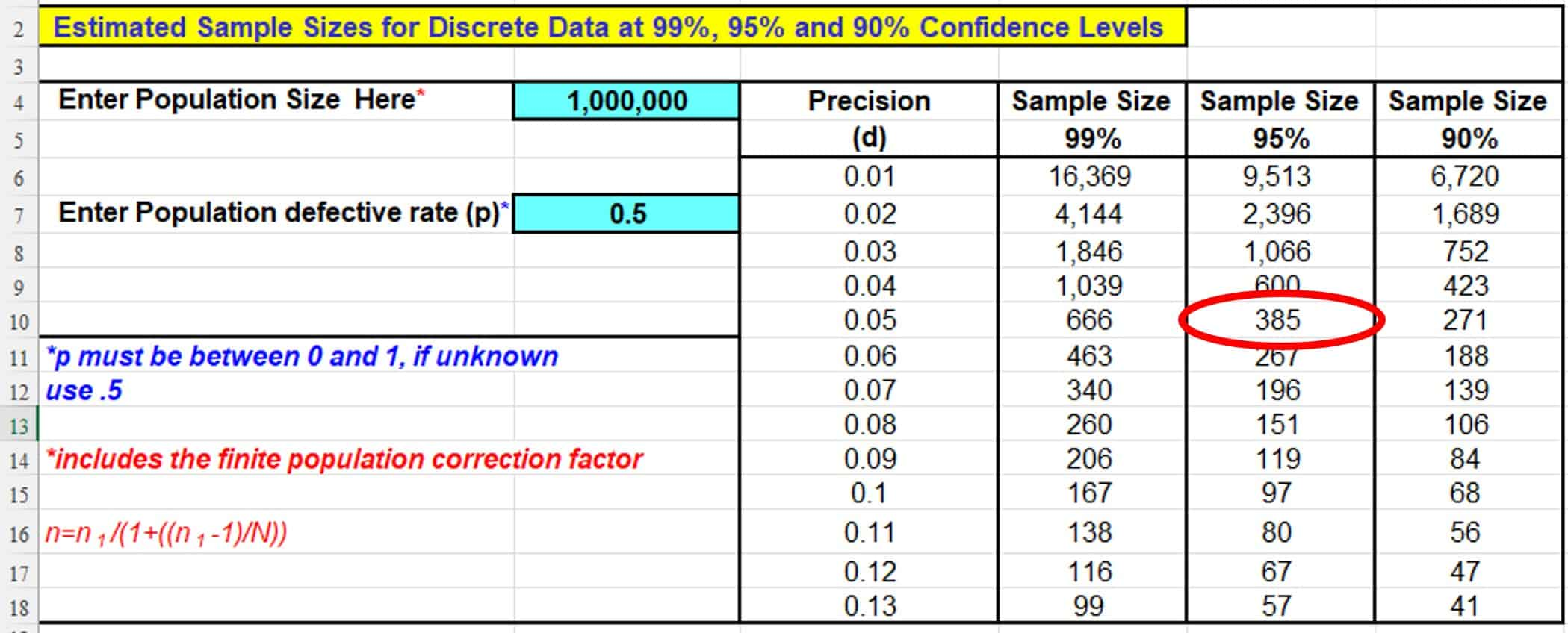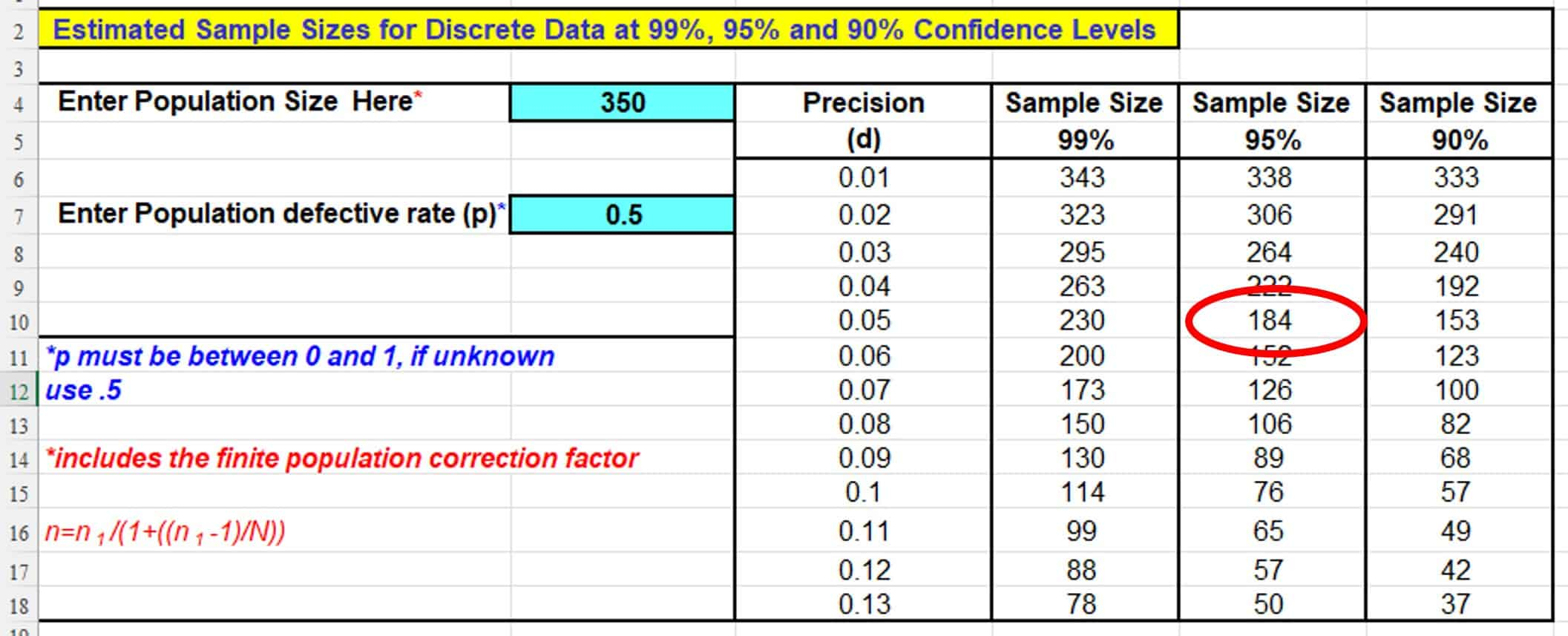Frequently Asked Questions (FAQ) about a sample size calculator

### What are the factors that are used for calculating sample size in a sample size calculator?

The factors are desired confidence level, degree of precision, estimated variation of the population and the size of the population.

### What is the formula for computing sample size for continuous data?

Sample size calculators will use the following formula for calculating sample size for continuous data:

### What if my calculated sample size is larger than my population?

If your sample size calculator indicates you should collect a sample larger than your entire population, you will need to adjust the calculated sample size by applying the Finite Population Correction Factor. This will allow you to reduce the required sample size based on the smaller size of the population.